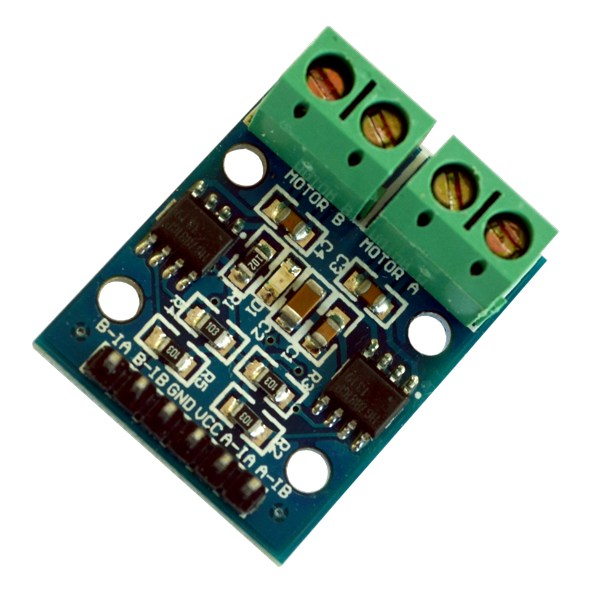Moonbase Otago - Dunedin Arduino project - Motor Controller

# Using a motor controller

## HG7881 motor controller

(Note: motor controllers are not included in the kit, Paul has a few, please ask if you think you might be able to use one)

We're using the HG7881 motor controllers mostly because they are cheap ... about a dollar each. They drive a pair of small electric motors forwards and backwards.Each has two L9110 h-bridge chips on it, one for each motor you can find a data sheet for this chip here.

An L9110 has two control inputs IA and IB and two outputs OA and OB which are connected to the motor it controlls. IA and IB can be connected to Arduino digital output pins to control them you program this way:.

 IA IB OA OB Action 0 0 0 0 stop 1 0 1 0 forwards 0 1 0 1 backwards 1 1 0 0 stop

The HG7881 board has two sets of connectors: the green screw connectors are for motors, the black 6 pin connector is for connecting up power and control from your Arduino.

The A-IA and A-IB pins are the IA and IB pins for motor A, the B-IA and B-IB pins are the IA and IB pins for motor B. GND is the ground pin and VCC is the power pin. You can drive VCC with up to 12 volts, normally you'd connect it to the same power switch the Arduino's external power suply is driven from.

## Programming the HG7881 motor controller

Here's some simple code for driving an HG7881. First let's define some output pins that are connected to the board:

```	const int left_A = D3;	// A-IA
const int left_B = D4;	// A-IB
const int right_A = D5;	// B-IA
const int right_B = D6;	// B-IB

void setup()
{
pinMode(left_A, OUTPUT);
pinMode(left_B, OUTPUT);
pinMode(right_A, OUTPUT);
pinMode(right_B, OUTPUT);
}
```

We can stop a motor by setting it's control pins to 0:

```	void
left_stop()
{
analogWrite(left_B, 0);
analogWrite(left_A, 0);
}

void
right_stop()
{
analogWrite(right_B, 0);
analogWrite(right_A, 0);
}

void
all_stop()
{
left_stop();
right_stop();
}
```

We use analogWrite() rather than digitalWrite() so we can control the motors' speed. We go forwards by holding the B input at 0 and driving the A input, and backwards by holding A at 0 and driving N:

```	void
left forward(int speed)
{
analogWrite(left_A, speed);
analogWrite(left_B, 0);
}

void
left_back(int speed)
{
analogWrite(left_B, speed);
analogWrite(left_A, 0);
}

void
right_forward(int speed)
{
analogWrite(right_A, speed);
analogWrite(right_B, 0);
}

void
right_back(int speed)
{
analogWrite(right_B, speed);
analogWrite(right_A, 0);
}

void
forwards(int speed)
{
left_forward(speed);
right_forward(speed);
}

void
back(int speed)
{
left_back(speed);
right_back(speed);
}
```

Finally we can write code to rotate in place, or to turn by braking one wheel with:

```	void rotate_left(int speed)
{
left_back(speed);
right_forward(speed);
}

void rotate_right(int speed)
{
left_forward(speed);
right_back(speed);
}

void turn_left(int speed)
{
left_stop();
right_forward(speed);
}

void turn_right(int speed)
{
left_forward(speed);
right_stop();
}

```